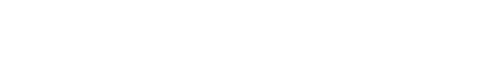# [RFC-0018] Proof of Stake reward adjustment

A task with many unknowns and variables, but with this solution many unpleasant sides and moments are eliminated.

I want to understand now the participation rate affects the inflation/reward rate. I understand that 100% minting means a full 3% (plus the “flat” rate of 0.25%)

If the minting participation rate is 10%, 25% or 50%, is there a predictable or set relationship to the inflation/reward? So that 10% participation means exactly 0.3% inflation, 25% participation is 0.75% inflation, and 50% participation is 1.5% inflation (plus the “flat” rate of 0.25%).

Or is the inflation occurring with sub-100% participation rate more variable, so that it averages out within a range?

Your numbers are right, it’s a linear relationship, so 10% participation is (3×0.1+0.25)%=0.55% pos inflation. For 25% participation its (3x0.25+0.25)%=1% pos inflation. However, the concept of participation is not clearly defined in this version, as we no longer have a coinage consumed sum. So the comparison is only found in post analysis (and when speaking theoretically). It is easier to simply track the inflation rate as a phenomenological number. Ideally, it would be best to separate the pow and pos components of the inflation rate (@willy) and compare them.

Why isn’t a slimmer ratio used, such as 2.5%/0.5% - this would cap inflation slightly lower, but would push the flat rate up. The RFC gives the following explanation, but I don’t really understand it. Thanks.

This ratio of 12:1 can be taken approximately as the continuous participation level (8.33%) below which stake grinding to dust values becomes profitable.

This is amazing, thank you.

I should mention that while rfc18 is just a x3+0.25 to the curve willy shows, rfc17 will reduce the curve in a non-trivial manner that is hard to capture.

The difference can be seen in the inverse of these two images (taken from the rfc11 thread in units of ‘adjustment’, which is essentially defined as inverse participation in rfc11):

Blue is without rfc17 and red is with rfc17. As you can see, the coinage limitation affected the curve at it’s most extreme value, reducing the estimated ‘participation’ from ~66% to ~50%.

Let us assume only 10% of coins are minting, which is a 0.55% inflation/minting reward. Unlike V0.8, that is 0.55% inflation of the entire money supply, not just the coins minting. So, the inflation/reward for the 10% that do mint will be 5.5%?

And if 25% of coins mint, that will be 1% total inflation, and so will represent a 4% reward for the minters of that 25%. And a 35% mint rate will be 1.3% inflation, and thus a reward for minters of 3.71% - and so on?

I believe the part you are seeing here is the supply-based component. The difficulty here is that we have to use a different definition of ‘participation’ to truly understand what happens. If a minter only mints periodically once a year, then they get almost nothing from the supply-based component. The continuous participation that shares the supply-based component is always less than the total participation responsible for the rated component, sometimes significantly so. In the current state of the chain, I estimate we have about 15% periodic participation and 10% continuous participation (for a total of 25% ‘participation’). This means that the total PoS inflation will be ~1%, while periodic minters are earning ~3% and continuous minters are earning ~5.5%. So to sum that up:
1% = 0.15x3% + 0.1x5.5%